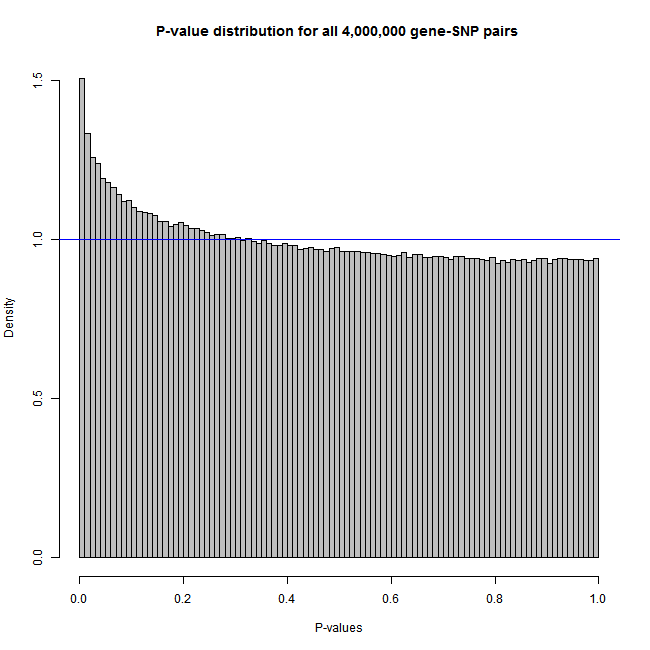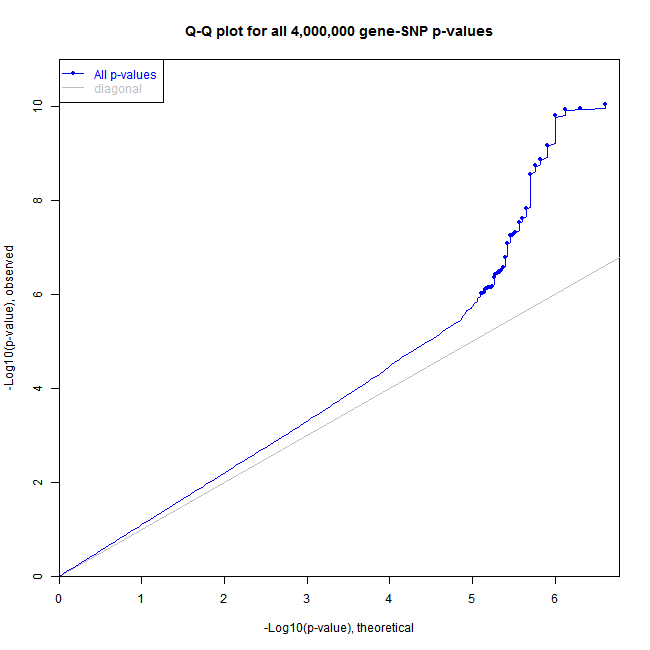﻿ Matrix eQTL, R sample code and dataset

# Matrix eQTL sample code

Package contains toy data set [data] and sample code [demo]: MatrixEQTL.tar.gz

The toy dataset consists of five files: genotype `SNP.txt`, expression `GE.txt`, a file `Covariates.txt` with two covariates, gender and age, and files `geneloc.txt` and `snpsloc.txt` with gene and SNP location information. First three files contain artificial information for 16 samples. The sample genotype file contains random values 0, 1, and 2 although the input genotype file is allowed to contain any real values.

## Test all gene-SNP pairs and plot a histogram of all p-values

`# Matrix eQTL by Andrey A. Shabalin# http://www.bios.unc.edu/research/genomic_software/Matrix_eQTL/# # Be sure to use an up to date version of R and Matrix eQTL.# source("Matrix_eQTL_R/Matrix_eQTL_engine.r");library(MatrixEQTL)## Location of the package with the data files.base.dir = find.package('MatrixEQTL');# base.dir = '.';## Settings# Linear model to use, modelANOVA, modelLINEAR, or modelLINEAR_CROSSuseModel = modelLINEAR; # modelANOVA, modelLINEAR, or modelLINEAR_CROSS# Genotype file nameSNP_file_name = paste(base.dir, "/data/SNP.txt", sep="");# Gene expression file nameexpression_file_name = paste(base.dir, "/data/GE.txt", sep="");# Covariates file name# Set to character() for no covariatescovariates_file_name = paste(base.dir, "/data/Covariates.txt", sep="");# Output file nameoutput_file_name = tempfile();# Only associations significant at this level will be savedpvOutputThreshold = 1e-2;# Error covariance matrix# Set to numeric() for identity.errorCovariance = numeric();# errorCovariance = read.table("Sample_Data/errorCovariance.txt");## Load genotype datasnps = SlicedData\$new();snps\$fileDelimiter = "\t";      # the TAB charactersnps\$fileOmitCharacters = "NA"; # denote missing values;snps\$fileSkipRows = 1;          # one row of column labelssnps\$fileSkipColumns = 1;       # one column of row labelssnps\$fileSliceSize = 2000;      # read file in slices of 2,000 rowssnps\$LoadFile(SNP_file_name);## Load gene expression datagene = SlicedData\$new();gene\$fileDelimiter = "\t";      # the TAB charactergene\$fileOmitCharacters = "NA"; # denote missing values;gene\$fileSkipRows = 1;          # one row of column labelsgene\$fileSkipColumns = 1;       # one column of row labelsgene\$fileSliceSize = 2000;      # read file in slices of 2,000 rowsgene\$LoadFile(expression_file_name);## Load covariatescvrt = SlicedData\$new();cvrt\$fileDelimiter = "\t";      # the TAB charactercvrt\$fileOmitCharacters = "NA"; # denote missing values;cvrt\$fileSkipRows = 1;          # one row of column labelscvrt\$fileSkipColumns = 1;       # one column of row labelsif(length(covariates_file_name)>0) { cvrt\$LoadFile(covariates_file_name);}## Run the analysisme = Matrix_eQTL_engine( snps = snps, gene = gene, cvrt = cvrt, output_file_name = output_file_name, pvOutputThreshold = pvOutputThreshold, useModel = useModel, errorCovariance = errorCovariance, verbose = TRUE, pvalue.hist = TRUE, min.pv.by.genesnp = FALSE, noFDRsaveMemory = FALSE);unlink(output_file_name);## Results:cat('Analysis done in: ', me\$time.in.sec, ' seconds', '\n');cat('Detected eQTLs:', '\n');show(me\$all\$eqtls)## Plot the histogram of all p-valuesplot(me)`

## Test local and distand gene-SNP pairs separately and plot Q-Q plots of local and distant p-values

`# Matrix eQTL by Andrey A. Shabalin# http://www.bios.unc.edu/research/genomic_software/Matrix_eQTL/# # Be sure to use an up to date version of R and Matrix eQTL.# source("Matrix_eQTL_R/Matrix_eQTL_engine.r");library(MatrixEQTL)## Location of the package with the data files.base.dir = find.package('MatrixEQTL');# base.dir = '.';## Settings# Linear model to use, modelANOVA, modelLINEAR, or modelLINEAR_CROSSuseModel = modelLINEAR; # modelANOVA, modelLINEAR, or modelLINEAR_CROSS# Genotype file nameSNP_file_name = paste(base.dir, "/data/SNP.txt", sep="");snps_location_file_name = paste(base.dir, "/data/snpsloc.txt", sep="");# Gene expression file nameexpression_file_name = paste(base.dir, "/data/GE.txt", sep="");gene_location_file_name = paste(base.dir, "/data/geneloc.txt", sep="");# Covariates file name# Set to character() for no covariatescovariates_file_name = paste(base.dir, "/data/Covariates.txt", sep="");# Output file nameoutput_file_name_cis = tempfile();output_file_name_tra = tempfile();# Only associations significant at this level will be savedpvOutputThreshold_cis = 2e-2;pvOutputThreshold_tra = 1e-2;# Error covariance matrix# Set to numeric() for identity.errorCovariance = numeric();# errorCovariance = read.table("Sample_Data/errorCovariance.txt");# Distance for local gene-SNP pairscisDist = 1e6;## Load genotype datasnps = SlicedData\$new();snps\$fileDelimiter = "\t";      # the TAB charactersnps\$fileOmitCharacters = "NA"; # denote missing values;snps\$fileSkipRows = 1;          # one row of column labelssnps\$fileSkipColumns = 1;       # one column of row labelssnps\$fileSliceSize = 2000;      # read file in slices of 2,000 rowssnps\$LoadFile(SNP_file_name);## Load gene expression datagene = SlicedData\$new();gene\$fileDelimiter = "\t";      # the TAB charactergene\$fileOmitCharacters = "NA"; # denote missing values;gene\$fileSkipRows = 1;          # one row of column labelsgene\$fileSkipColumns = 1;       # one column of row labelsgene\$fileSliceSize = 2000;      # read file in slices of 2,000 rowsgene\$LoadFile(expression_file_name);## Load covariatescvrt = SlicedData\$new();cvrt\$fileDelimiter = "\t";      # the TAB charactercvrt\$fileOmitCharacters = "NA"; # denote missing values;cvrt\$fileSkipRows = 1;          # one row of column labelscvrt\$fileSkipColumns = 1;       # one column of row labelsif(length(covariates_file_name)>0) { cvrt\$LoadFile(covariates_file_name);}## Run the analysissnpspos = read.table(snps_location_file_name, header = TRUE, stringsAsFactors = FALSE);genepos = read.table(gene_location_file_name, header = TRUE, stringsAsFactors = FALSE);me = Matrix_eQTL_main( snps = snps, gene = gene, cvrt = cvrt, output_file_name     = output_file_name_tra, pvOutputThreshold     = pvOutputThreshold_tra, useModel = useModel, errorCovariance = errorCovariance, verbose = TRUE, output_file_name.cis = output_file_name_cis, pvOutputThreshold.cis = pvOutputThreshold_cis, snpspos = snpspos, genepos = genepos, cisDist = cisDist, pvalue.hist = "qqplot", min.pv.by.genesnp = FALSE, noFDRsaveMemory = FALSE);unlink(output_file_name_tra);unlink(output_file_name_cis);## Results:cat('Analysis done in: ', me\$time.in.sec, ' seconds', '\n');cat('Detected local eQTLs:', '\n');show(me\$cis\$eqtls)cat('Detected distant eQTLs:', '\n');show(me\$trans\$eqtls)## Plot the Q-Q plot of local and distant p-valuesplot(me)`

## Create an artificial dataset and plot the histogram and Q-Q plot of all p-values

`library('MatrixEQTL')# Number of samplesn = 100;# Number of variablesngs = 2000;# Common signal in all variables (population stratification)pop = 0.2 * rnorm(n);# data matricessnps.mat = matrix(rnorm(n*ngs), ncol = ngs) + pop;gene.mat = matrix(rnorm(n*ngs), ncol = ngs) + pop + snps.mat*((1:ngs)/ngs)^9/2;# data objects for Matrix eQTL enginesnps1 = SlicedData\$new( t( snps.mat ) );gene1 = SlicedData\$new( t( gene.mat ) );cvrt1 = SlicedData\$new( );rm(snps.mat, gene.mat)# Slice data in blocks of 500 variablessnps1\$ResliceCombined(500);gene1\$ResliceCombined(500);# name of temporary output filefilename = tempfile();# Perform analysis recording information for # a histogrammeh = Matrix_eQTL_engine(  snps = snps1,  gene = gene1,  cvrt = cvrt1,  output_file_name = filename,   pvOutputThreshold = 1e-100,   useModel = modelLINEAR,   errorCovariance = numeric(),   verbose = TRUE,  pvalue.hist = 100);unlink( filename );# png(filename = "histogram.png", width = 650, height = 650)plot(meh, col="grey")# dev.off();``# Perform the same analysis recording information for # a Q-Q plotmeq = Matrix_eQTL_engine( snps = snps1, gene = gene1, cvrt = cvrt1, output_file_name = filename, pvOutputThreshold = 1e-6, useModel = modelLINEAR, errorCovariance = numeric(), verbose = TRUE, pvalue.hist = "qqplot");unlink( filename );# png(filename = "QQplot.png", width = 650, height = 650)plot(meq, pch = 16, cex = 0.7)# dev.off();`## Analyzing you own data

Please follow to Getting started with Matrix eQTL for information on how to apply Matrix eQTL to your own data.

By Andrey Shabalin